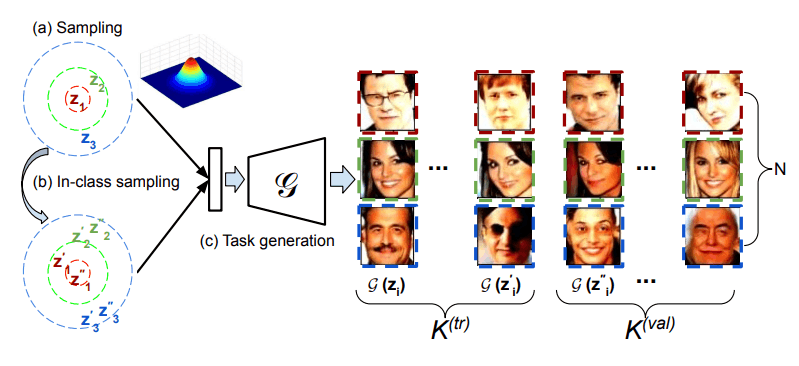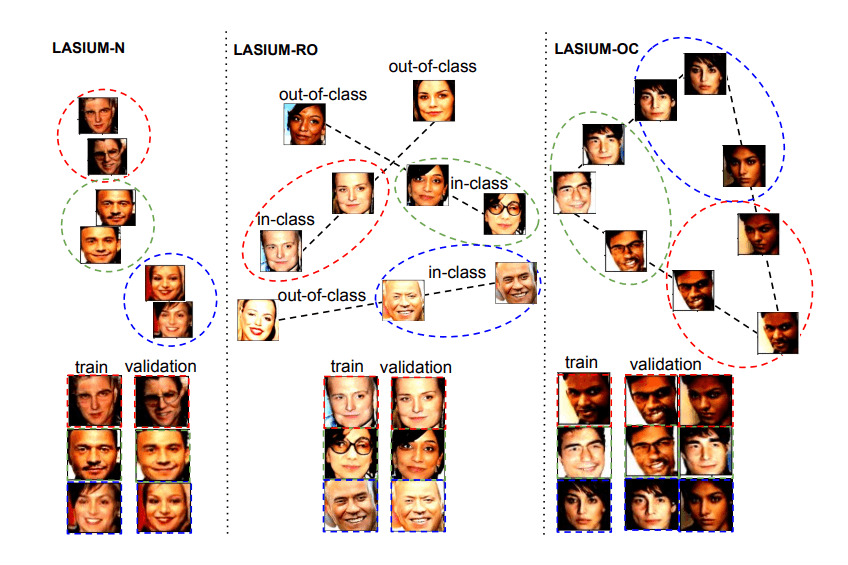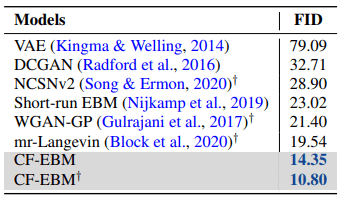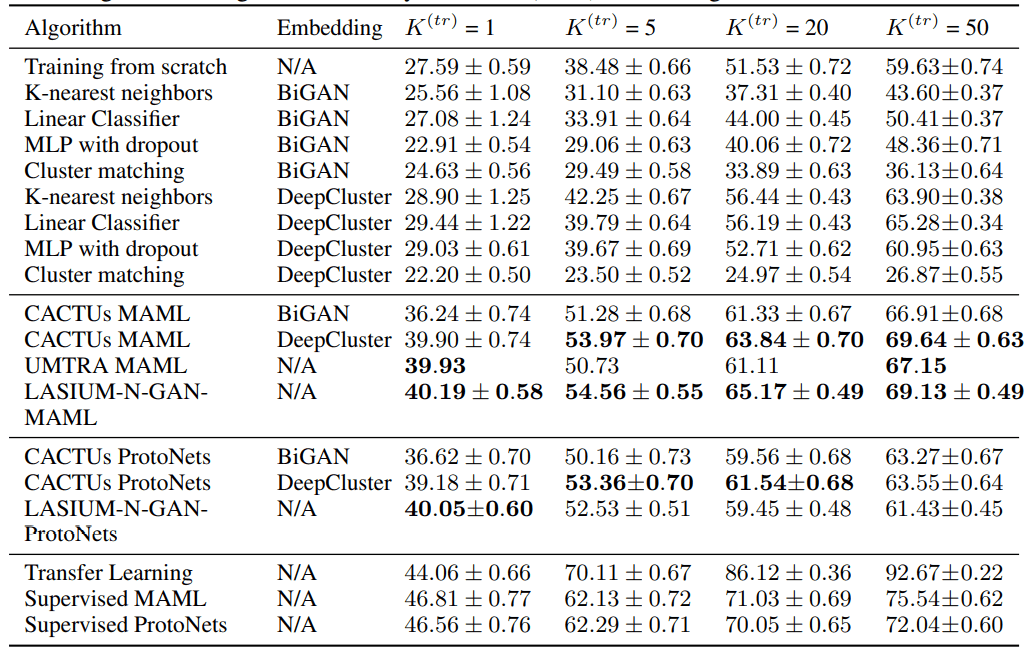# Catch up on the latest AI articles# The Potential Of Unsupervised Meta-learning Using Deep Generative Models [ICLR2021]

3 main points
✔️ Generate artificially labeled data in meta-tasks using linear interpolation in latent space
✔️ Proposed three methods (LASIUM-N, LASIUM-RO, LASIUM-OC) for data generation by linear interpolation
✔️ Outperformed conventional methods for unsupervised meta-learning and approached the performance of supervised meta-learning

(Submitted on 18 Jun 2020)

Subjects: Machine Learning (cs.LG); Machine Learning (stat.ML)code：The images used in this article are from the paper, the introductory slides, or were created based on them.

## What is meta-learning?

People can use their past experiences to learn new things. For example, a person who knows how to play chess can easily learn to play chess.

Meta-learning is the introduction of this kind of "learning how to learn" learning method to machine learning. In most cases, neural network learning starts with no prior knowledge, but in meta-learning, the accuracy of the target task is efficiently improved by solving related tasks that are different from the target task.

A similar approach to meta-learning is fine-tuning. In this approach, the network parameters obtained in the related task are used as initial values for solving the target task, so unlike meta-learning, performance improvement in the target task is not guaranteed.

### Unsupervised v.s. Supervised

Meta-learning can be broadly divided into "unsupervised" and "supervised" methods. Supervised meta-learning uses datasets that are explicitly labeled with relevant tasks. Unsupervised meta-learning, on the other hand, uses pseudo-labeled datasets generated by clustering or data expansion.

Although unsupervised meta-learning can train in areas where labels are not readily available and offer advantages in dataset size over supervised meta-learning, its performance is mostly inferior to supervised meta-learning.

### Towards unsupervised meta-learning that is comparable to supervised

One of the reasons why unsupervised meta-learning is inferior to supervised meta-learning is the low accuracy of pseudo-labels. In the setting where there is no teacher label, the accuracy of the label given by clustering directly affects the performance.

In this paper, we address this problem by using a data set for learning-related tasks. Using data sampled from the latent space of a deep generative model We address this problem by

## Generating meta-learning tasks using deep generative models.

This section describes the proposed method of this paper, LASIUM (LAtent Space Interpolation Unsupervised Meta-learning).

The difficulty in unsupervised meta-learning lies in how to generate a task from an unlabeled data set. Assuming that the task is an N-class classification problem, we need to prepare K samples for each class for training and validation. To obtain these samples, conventional methods either sample directly from the data set or perform data expansion. On the other hand, in the method introduced here, it is possible to train using data not included in the data set by sampling after training the data generation model to the neural network.

The following figure illustrates the flow of generating a meta-learning task (3-class classification) using GAN. Initially, we train a deep generative model using an unlabeled data set. Next, we sample the data belonging to different classes. This corresponds to sampling $z_1,z_2,z_3$ from the latent space and mapping them to the data space using the generator. (a) In addition, data belonging to the same class as the one just sampled are obtained by the same procedure. ($z'$ in the figure ) (b ) Finally, the data obtained in the above manner is divided into two parts, one for training and the other for evaluation, and a meta-task is generated. (c)The following sections describe each step in detail.

### (1) Training of deep generative models

Initially, we train a neural network with a generative model $p(x)$ for an unlabeled data set. MSGAN and PGGAN, which are derivatives of VAE and GAN, are chosen as such deep learning models.

MSGAN is a model that adds a regularization term for mode decay, and PGGAN is a model that adds neural network layers step by step according to the learning progress of GAN.

### (2) Sampling of different class data

To prepare (N x K) data required for the N-class classification problem, we sample one starting data (anchor) for each class. in GAN. In VAE, we use an encoder to sample the data from the dataset such that the pairwise distance is greater than or equal to the threshold $\epsilon$ when mapping to the latent space.

### (3) Sampling of same class data

After sampling the anchor vectors, we go on to sample the data that has a latent representation close to each anchor. By doing so, we can obtain data that seems to belong to the same class as each anchor. There are three methods proposed in this paper and linear interpolation in latent space is used.

LASIUM-N

It is a method to obtain the same class data by adding Gaussian noise to the anchor vector and then mapping it to the data space.

LASIUM-RO

Randomly sample hetero lass vectors $\mathbb{v}$ that are more than $\epsilon$ away from the anchor vector, and compute the home class vector $\mathbb{z}$ by linear interpolation between the anchor vector $\mathbb{z}$ and the hetero lass vector $\mathbb{v '}$ is calculated. The method then maps the latent space to the data space to obtain the same class data.

In the linear interpolation formula $\mathbb{z'} = \mathbb{z} + \alpha \times (\mathbb{v - z})$, you can adjust the closeness to the anchor vector by changing the hyperparameter $\alpha$.

LASIUM-OC

LASIUM-RO is a method to calculate the same class vector by linear interpolation between the anchor vectors of one class and those of a different class and to obtain the same class data by mapping to the data space. It is the same as LASIUM-RO in that it obtains the same class data by linear interpolation between different class vectors, but it differs in that it selects the different class data randomly or from anchors.

The figure below illustrates the differences in sampling methods for the same class data. The colored dotted lines indicate each class, and the gray dotted lines in LASIUM-RO and LASIUM-OC indicate the vector $(\mathbb{v - z})$ in linear interpolation.## data set

We checked the performance of the above algorithms on four different few-shot learning benchmarks. On a test dataset, we evaluated the model by computing its accuracy on a meta-learning task generated by the deep generative model described above. In this blog, we will only deal with results 2 and 4.

1. Omniglot: Five-class classification in handwritten character recognition datasets.
2. CelebA: Five-class classification in-person recognition datasets.
3. CelebA attributes a dataset of binary labels annotated concerning facial features.
4. mini-ImageNet: A dataset containing 100 randomly selected classes from ImageNet ILSVRC-2012.

## experimental results

### CelebA

The table below shows the evaluation results for the task of person recognition (5-class classification) in CelebA. The number of training samples used for one class is either {1, 5, 15}, and is indicated as $K^{(tr)}$ in the table. The number of samples for evaluation is fixed at 15. The numbers in the table represent the average performance of 1000 tasks sampled for evaluation, with 95% confidence intervals.As can be seen from the table, although the proposed method is inferior to supervised meta-learning, it is more accurate than the unsupervised meta-learning comparison methods, CACTUs and UMTRA. It can be seen that the proposed method has a small performance drop when the number of training data samples is small.

### miniImageNet

The table below shows the results of our evaluation on the task of five-class classification in miniImageNet. The number of training samples used per class is either {1,5,20,50} and is denoted as $K^{(tr)}$ in the table. The number of samples for evaluation is fixed at 15. The numbers in the table are the average performance of 1000 tasks sampled for the evaluation, and the 95% confidence intervals are given.

The bottom eight algorithms in the top row are evaluated using embedded representations obtained by unsupervised learning, the nine algorithms in the middle two rows are evaluated for unsupervised meta-learning, and the three algorithms in the bottom row are evaluated for transfer learning and supervised meta-learning.As can be seen from the above table, the accuracy of the unsupervised meta-learning method is lower than that of the supervised learning method, but it records a higher percentage of correct answers than the mere unsupervised method. In addition, the proposed method always has the top3 accuracy in unsupervised meta-learning methods, which indicates that the range of accuracy decrease is small when the number of training samples is small.

## in the end

We can say that LASIUM is more suitable for the setting of Few-Shot Learning because it does not lose accuracy even when the number of training data in each class is small. By learning the generative model of the data first, it is effective to sample a variety of data without complicated data expansion.

I'll be keeping an eye on future developments in meta-learning research!

If you have any suggestions for improvement of the content of the article,